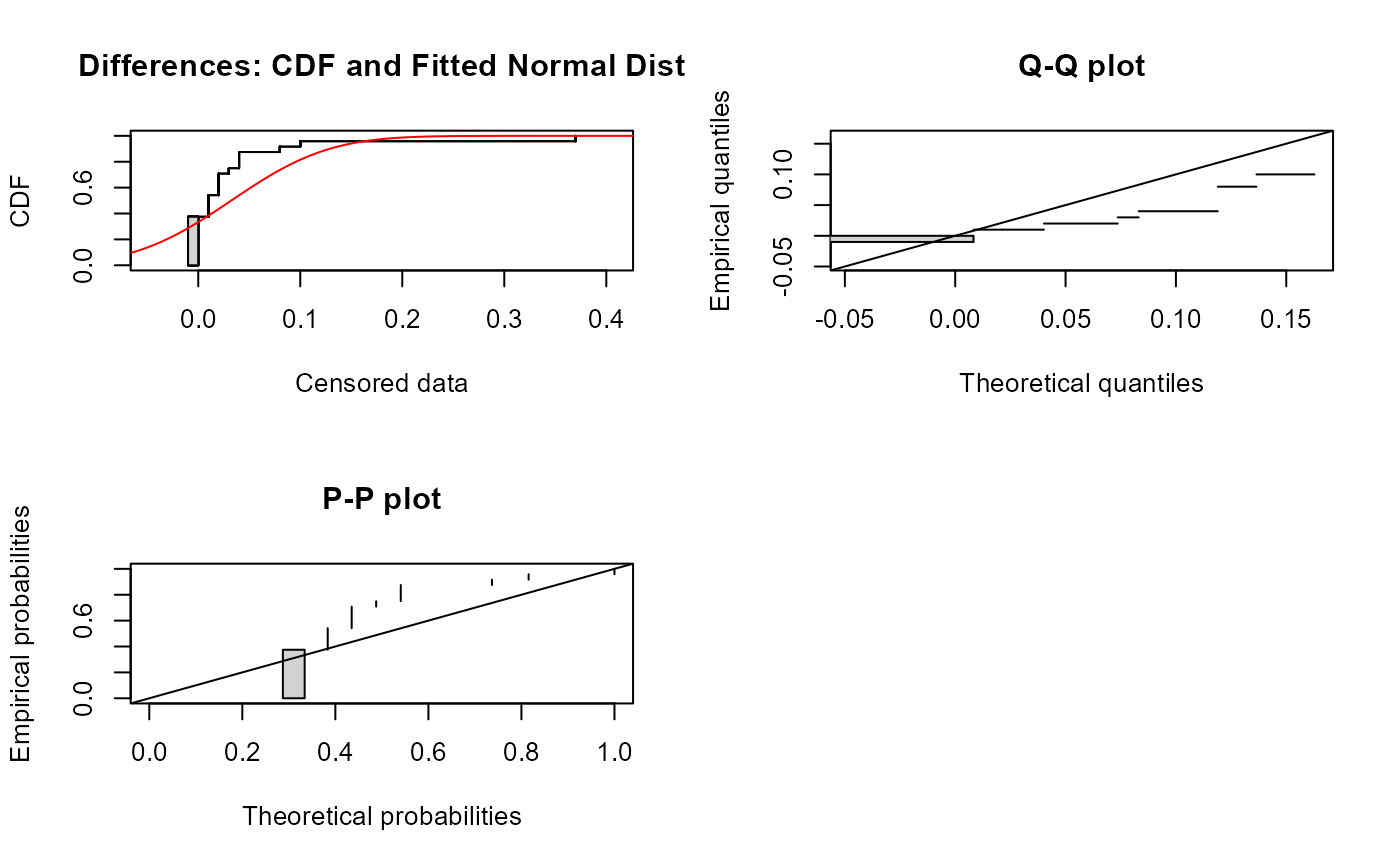Performs a parametric test of whether the mean difference between two columns of paired censored data equals 0. Assumes that the paired differences follow a gaussian (normal) distribution.

cen_paired(xd, xc, yd, yc, alternative = "two.sided", printstat = TRUE)

## Arguments

xd

The first column of data values plus detection limits

xc

The column of censoring indicators, where 1 (or TRUE) indicates a detection limit in the xd column, and 0 (or FALSE) indicates a detected value in xd.

yd

The second column of data values plus detection limits, or a single number representing a standard / guideline value.

yc

The column of censoring indicators for yd, where 1 (or TRUE) indicates a detection limit in the yd column, and 0 (or FALSE) indicates a detected value in yd. Not needed if yd is a single standard number.

alternative

The usual notation for the alternate hypothesis. Default is “two.sided”. Options are “greater” or “less”.

printstat

Logical TRUE/FALSE option of whether to print the resulting statistics in the console window, or not. Default is TRUE.

## Value

A list of statistics containing the following components:

• n Number of observations

• Z The value of the test statistic

• p.value the p-value of the test

• Mean difference the mean difference between xd and yd

## Details

You may also test for whether the mean of the xd data exceeds a standard by entering the single number for the standard as yd. In that case no yc is required.

## Examples


data(atrazine)

cen_paired(atrazine$June,atrazine$JuneCen,atrazine$Sept,atrazine$SeptCen)
#> Censored paired test for mean(atrazine$June - atrazine$Sept) equals 0.
#>  alternative hypothesis: true mean difference does not equal 0.
#>
#>  n = 24   Z= -1.0924   p-value = 0.2747
#>  Mean difference = -3.927# Comparing standard/guieline value
cen_paired(atrazine$June, atrazine$JuneCen, 0.01, alternative = "greater")
#>  Censored paired test for mean(atrazine$June) equals 0.01 #> alternative hypothesis: true mean atrazine$June exceeds 0.01.
#>
#>  n = 24   Z= 2.1004   p-value = 0.01785
#>  Mean atrazine\$June = 0.04231